Function Repository Resource:

# MinkowskiSausageCurve

Generate the Minkowski sausage curve

Contributed by: Sander Huisman
 ResourceFunction["MinkowskiSausageCurve"][n] generates the line segments of the Minkowski sausage curve with n iterations. ResourceFunction["MinkowskiSausageCurve"][{p1,p2},n] uses the line connecting the points p1 and p2 as the initial line. ResourceFunction["MinkowskiSausageCurve"][{p1,p2,…,pn},n] uses the lines joining the points pi.

## Details

The following transformation is done with each line segment: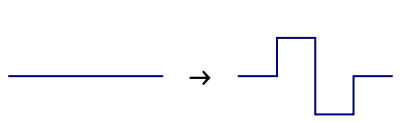The first four iterations: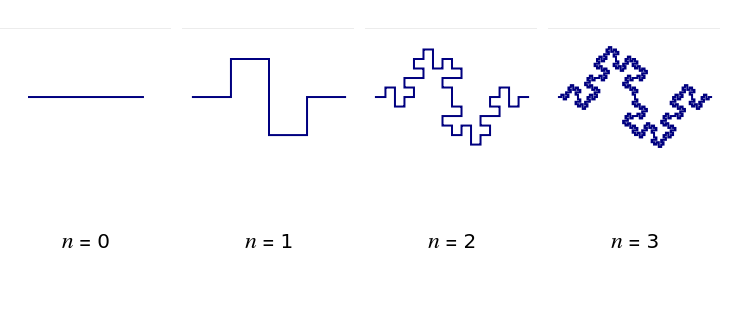ResourceFunction["MinkowskiSausageCurve"][n] is equivalent to ResourceFunction["MinkowskiSausageCurve"][{{-1,0},{1,0}},n].
The syntax ResourceFunction["MinkowskiSausageCurve"][Line[{p1,p2,,pn}],n] is also supported.

## Examples

### Basic Examples (2)

Generate the Minkowski sausage curve for two iterations:

 In:=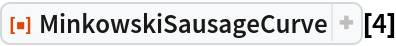Out=Generate the curve for four iterations and visualize the result:

 In:=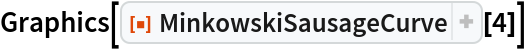Out=### Scope (1)

Start from a square:

 In:=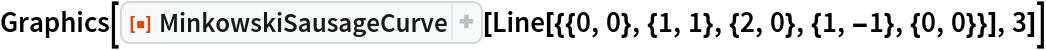Out=### Properties and Relations (2)

MinkowskiSausageCurve and KochCurve have identical first iterates:

 In:=Out=Succeeding iterates have different behavior:

 In:=Out=The number of elements grows exponentially:

 In:=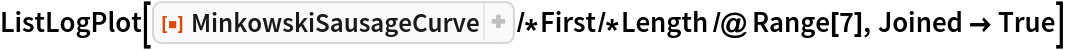Out=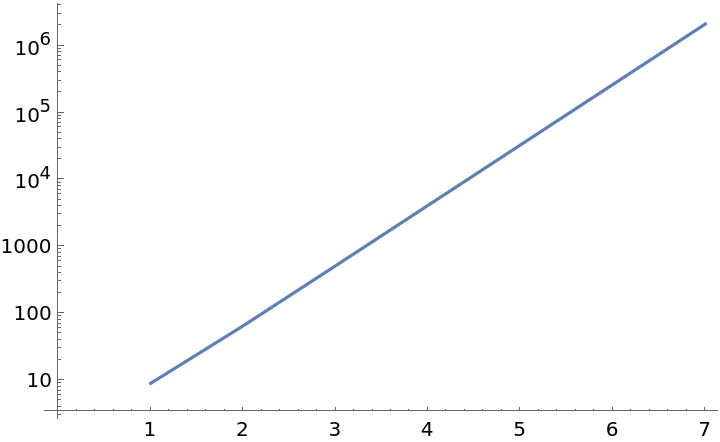SHuisman

## Version History

• 1.0.0 – 28 October 2021